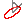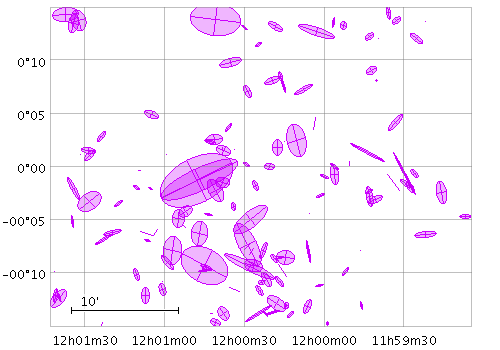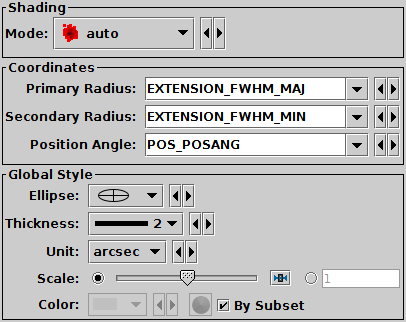Next Previous Up Contents
Next: XYCorr Form
Up: Plot Forms
Previous: XYEllipse Form

#### A.4.5.8 SkyEllipse Form

The SkyEllipse () form plots an ellipse (or rectangle, triangle, or other similar figure) defined by two principal radii and an optional angle of rotation, the so-called position angle. This angle, if specified, is in degrees and gives the angle from the North pole towards the direction of increasing longitude on the longitude axis.Example SkyEllipse plotSkyEllipse form configuration panel

The configuration options are:

The two principal radii for the ellipse. If auto-scaling is off, these are in units of degrees. It doesn't matter whether the primary is larger than the secondary. If the Secondary Radius field is left blank, it is assumed to equal the Primary Radius field, i.e. the ellipses are circles.
Position Angle
Orientation of the ellipse. This is the angle in degrees from the North pole to the primary axis of the ellipse in the direction of increasing longitude. If the value is missing (either this field not filled in or blank in the data) it is considered to be zero.
Ellipse
Ellipse graphical representation, selected from a range of options (includes also rectangles, crosses etc).
Thickness
Controls the line thickness used when drawing ellipses. Zero, the default value, means a 1-pixel-wide line is used. Larger values make drawn lines thicker, but note changing this value will not affect all shapes, for instance filled ellipses contain no line drawings.
Scale
Changes the factor by which all ellipse sizes are scaled. If the ellipses are too small, slide it right, if they are too big, slide it left. The slider scale is logarithmic. Alternatively, enter a fixed value in the text field.
Auto Scale
If selected, this option will determine the default ellipse scale size from the data - it will fix it so that the largest ellipses are a few tens of pixels long by default. That scaling can then be adjusted using the Scale slider. If unselected, then the default position of the Scale slider corresponds to the actual values in degrees given by the submitted ellipse radii coordinates.

Next Previous Up Contents
Next: XYCorr Form
Up: Plot Forms
Previous: XYEllipse Form

TOPCAT - Tool for OPerations on Catalogues And Tables